Reach Us+44-1904-929220
Combined Effects of Radiation and Oblateness on the Existence and Stability of Equilibrium Points in the Perturbed Restricted Four-Body Problem | OMICS International
Journal of Astrophysics & Aerospace Technology
All submissions of the EM system will be redirected to Online Manuscript Submission System. Authors are requested to submit articles directly to Online Manuscript Submission System of respective journal.

Combined Effects of Radiation and Oblateness on the Existence and Stability of Equilibrium Points in the Perturbed Restricted Four-Body Problem

Singh J and Vincent AE*

Department of Mathematics, Faculty of Science, Ahmadu Bello University, Zaria, Nigeria

*Corresponding Author:
Vincent Aguda Ekele
Department of Mathematics, Faculty of Science
Ahmadu Bello University, Zaria, Nigeria
Tel: +603) 6196 4053;
E-mail: [email protected]

Received Date: June 21, 2016; Accepted Date: March 15, 2017; Published Date: February 21, 2017

Citation: Singh J, Vincent AE (2017) Combined Effects of Radiation and Oblateness on the Existence and Stability of Equilibrium Points in the Perturbed Restricted Four-Body Problem. J Astrophys Aerospace Technol 5:139. doi: 10.4172/2329- 6542.1000139

Copyright: © 2017 Singh J, et al. This is an open-access article distributed under the terms of the Creative Commons Attribution License, which permits unrestricted use, distribution, and reproduction in any medium, provided the original author and source are credited.

Visit for more related articles at Journal of Astrophysics & Aerospace Technology

Abstract

We study numerically the perturbed problem of four bodies, where an infinitesimal body is moving under the Newtonian gravitational attraction of three much bigger bodies (called the primaries). The three bodies are moving in circles around their centre of mass fixed at the origin of the coordinate system, according to the solution of Lagrange where they are always at the apices of an equilateral triangle. The fourth body does not affect the motion of the primaries. The problem is perturbed in the sense that the dominant primary body m1 is a radiation source while the second smaller primary m2 is an oblate spheroid, with masses of the two small primaries m2 and m3 taken to be equal. The aim of this study is to investigate the effects of radiation and oblateness parameters on the existence and location of equilibrium points and their linear stability. It is observed that under the perturbative effect of oblateness, collinear equilibrium points do not exist (numerically and of course analytically) whereas the positions of the noncollinear equilibrium points are affected by the radiation and oblateness parameters. The stability of each equilibrium points ( Li,i = 1,...,6) is examined and we found that points Li,i = 1,...,6 are unstable while L7 and L8 are stable.

Keywords

Four-body problem; Radiation; Oblateness; Equilibrium points; Stability

Introduction

One of the most extensively studied problems in Celestial Mechanics is the restricted three-body problem (R3BP), where one of the bodies is considered massless; so, that it does not affect the motion of the other two that move in a Keplerian orbits. Restricted four-body problem (R4BP) is a modification of the R3BP and a natural generalization of it. It deals with the motion of an infinitesimal particle under the Newtonian gravitational attraction of three bodies, called primaries, whose trajectories are the solution of the three Newtonian body problems.

In the same direction as several restricted three-body problems have given much insight about real three-body problems, the study of special type of four-body problem may be useful to understand the dynamical behavior of stellar systems through analytical or numerical studies. It is worth to note that, the four-body problem is increasingly being used for explaining many complex dynamical phenomena that appear in the solar system and exoplanetary systems. The restricted four-body problem has many possible uses in the dynamical system. For example, the fourth body is very useful for saving fuel and time in the trajectory transfers . An application of the restricted four-body problem is also illustrated in the general behavior of the synchronous orbits in presence of the Moon as well as the Sun.

In recent time, many researchers have taken into account the effects of radiation and oblateness in their study of restricted threebody problem [2-9].

Similarly, the classical restricted four-body problems may be generalized to include different types of effects such as radiation pressure force, Poynting-Robertson drag, oblateness of the bodies, Coriolis and centrifugal forces, variation of the masses of the primaries, etc. It is known that in the general problem of three bodies there is a particular solution in which the bodies are placed at the vertices of an equilateral triangle, each moving in a Keplerian orbit. This is well known, and was first studied by Lagrange in 1772. He found a solution where the three bodies remain at constant distances from each other while they revolved around their common center of mass. There has been recently an increased interest for this model because of some observational evidence; as it is known the Sun, Jupiter and the Trojan asteroids formed such a configuration in our Solar system.

In this paper, we study the R4BP in which the primaries are in the Lagrange equilateral triangle configuration. In the framework of this model many works have been done in the last years. For example, Alvarez-Ramirez and Vidal  studied the equilateral R4BP in the case where the three primaries have equal masses. Baltagiannis and Papadakis  investigated the number of the equilibrium points of the problem for any value of the masses, and studied numerically their linear stability varying the values of the masses. Besides, they showed the regions of the basins of attraction for the equilibrium points for some values of the mass parameters. In Burgos-Garcia and Delgado , the authors studied the periodic orbits of the problem for the case of two equal masses approximately at Routh’s critical value. Recently, Papadouris and Papadakis  studied the equilibrium points in the photogravitational R4BP for the case of two equal masses. Also, the linear stability of each equilibrium point was examined. Kumari and Kushvah  studied the stability regions of the equilibrium points in the problem by taking into account the oblateness of the two small primaries. They established eight equilibrium points, two collinear and six non-collinear points and further observed that the stability regions of the equilibrium points expanded due to the presence of oblateness coefficients and various values of Jacobi constant, C. The allowed regions of motion of the infinitesimal body as well as the regions of the basins of attraction for the equilibrium points were given. More recently, Singh and Vincent  studied the out-of-plane equilibrium points in the photogravitational R4BP; however, they considered all the primary bodies as radiation sources with two of the bodies having the same radiation and mass value.

This model has been used for practical applications by some researchers in the last years. For example, Ceccaroni and Biggs  investigated the stability of the problem and tested the results in a real Sun-Jupiter- (624) Hektor-spacecraft system, Baltagiannis and Papadakis  studied periodic solutions of the problem in the Sun- Jupiter-Trojan Asteroid-spacecraft system.

In this paper, we extend the basic model of the restricted four-body problem by considering the dominant primary body m1 as a radiation source, while the second primary body m2 is modeled as oblate spheroid with the aim to investigate the changes which may result in these dynamical features due to the parameters of this model-problem. In particular, we study the existence and location of the equilibrium points as well as their linear stability under the effects of radiation and oblateness parameters.

The paper is organized as follows: Section 2 present the equations of motion and the Jacobi integral of the system. Section 3 determines the equilibrium points, while Section 4 investigates their linear stability. Section 5 discusses the obtained results and conclusion of the paper.

Equations of Motion

We consider that three bodies of masses m1 , m2 and m3 (m1 >> m2 = m3 ) always lie at the vertices of an equilateral triangle and one of them, say m1 , is on the positive x-axis at the origin of time (Figure 1). A fourth infinitesimal body, moving in the same plane, is acted upon the attraction of the three primary bodies. The motion of the system is referred to axes rotating with uniform angular velocity. The equilateral configuration is possible for all distributions of the masses, whilst the fourth body of negligible mass moves in the same plane. We suppose that the origin is taken as the center of gravity of the system and that the motion of the infinitesimal mass m is governed by the gravitational force of the primaries. We adopt the sum of the masses of the primaries and the distance between them as the units of mass and length. We choose the unit of time such as to make the gravitation constant equal to unity. Let the coordinates of the infinitesimal mass be (x, y) and those of masses m1 , m2 and m3 are: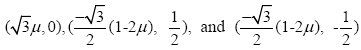,respectively, relative to a rotating frame of reference Oxy, where O is the origin,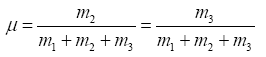is the mass parameter, where μ∈ (0,1/2). The perturbed mean motion n is given by n2= 1+3A2/2 where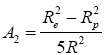is the oblateness coefficient of m2 with Re and Rp, as the equatorial and polar radii, respectively, and R is the separation between the primaries. In general, we have 0 < A2 << 1. The factor characterizing the radiation pressure of the dominant primary body m1 is expressed by means of the radiation factor q1 = 1-δ, where δ is the ratio of the force Fr which is caused by radiation to the force Fg which results from gravitation due to the primary body m1 .

The equations of motion of the infinitesimal mass in the rotating coordinate system are written as (see, for example, Papadouris and Papadakis,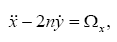(1)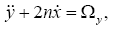(2)

where,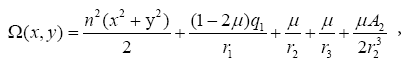(3)

with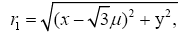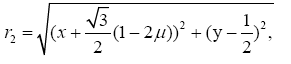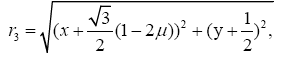Here r1, r2 and r3 are the distances of the infinitesimal body from the primaries, Ω is the gravitational potential, dots denote time derivatives, the suffixes x and y indicate the partial derivatives of Ω with respect to x and y, respectively.

It is well known that these equations have the so, called Jacobi first integral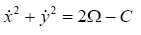(4)

where C is the Jacobian constant.

Linear stability of the Lagrange configuration

It is known, that in the Lagrange central configuration the necessary condition for the stability of the configuration is the inequality,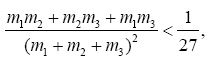(5)

where m1 , m2 and m3 are the three primary bodies . Later, Routh  studied the linear stability of the same solutions in the case of homogeneous potentials. Recently, Papadouris and Papadakis  produced the necessary condition for the stability of the Lagrange central configuration in the photogravitational R4BP based on the ideas of Santroprete  and Moeckel . Based on these ideas, if someone replaces the masses mi=qi(1-Ai) mi , i=1,2,3, then we believe that it will be produced the necessary condition for the stability of the Lagrange central configuration, in the present problem, i.e.,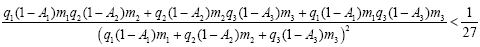(6)

where m1 , m2 and m3 are the three primary bodies, A1, A2and A3 are the oblateness factors of the primaries, respectively, and q1, q2and q3 are the corresponding radiation pressure forces of the primaries. Now, if we put q1 =q2 =q3 =1and A1 =A2 =A3 = 0, we obtain inequality (5) of the linear stability of the Lagrange configuration (Gascheau 1843). For q1 ≠1 and q2 =q3=1; A1 =A2 =A3 = 0,we obtain the photogravitational case of Papadouris and Papadakis.

In our present case where the dominant primary body m1 is a radiation source while the second smaller primary body m2 is an oblate spheroid, the problem admits inequality of the form,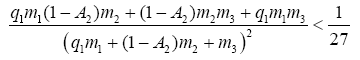(7)

In the gravitational case Baltagiannis and Papadakis,  where the two small primary bodies have the same mass, we know that only for a large value of m1and small masses m2 and m3 , the Gascheau’s inequality is fulfilled. In the case where the dominant primary body m1 is a radiation source while the other two small primaries have equal masses (m2 = m3 ), then a detailed study of the equilibrium points of the problem has been done by Papadouris and Papadakis . In present work, we will consider sets of (mi, A2, q1) which satisfy the condition (7). So, we assume that we have a dominant primary body with mass mi=0.98 and two small equal primaries with masses m2 = m3 =μ=0.01.

Location and Existence of the Equilibrium Points

The equilibrium points are those points at which the velocity and acceleration of the fourth body are zero. Therefore, the location of these points are given by the solutions of the equations,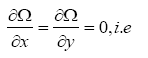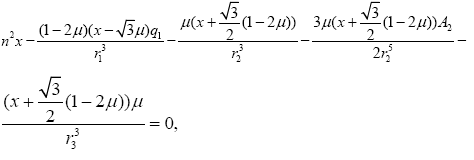(8)

and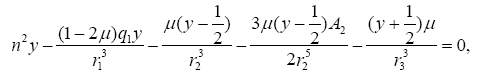(9)

Collinear equilibrium points

The collinear points are the solutions of Equations (8), (9) when y = 0. If, y = 0, Equation (9) is not fulfilled (since A2 ≠ 0). Thus, the solutions of Equation.(8) will not correspond to equilibrium points on the x-axis, called collinear equilibrium points. Hence, collinear equilibrium points do not exist (numerically and of course neither analytically) under the combine effects of radiation and oblateness of the dominant and small primaries, respectively. This is not strange because we can find in the literature that in the four-body problem and for example, when m1 ≠ m2 ≠ m3 (three different masses), then collinear equilibrium points do not exist (see for details Baltagiannis and Papadakis . So, in this problem there are cases where collinear equilibria do not exist.

Non-collinear equilibrium points

The non-collinear points can be found by solving Equations (8) and (9) simultaneously when y ≠ 0, that is,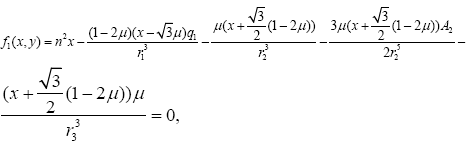(10)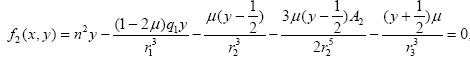(11)

and the problem, for μ=0.01, q1=0.99 and A2 = 0.01, admits eight noncollinear equilibrium points Li, i =1-8 (Figure 2). We opted to name them in the same way as in the work of Kumari and Kushvah . One can easily see eight points of intersection of the curves, which corresponds to eight equilibrium positions of the infinitesimal body m. The three large black points are the positions of the primary bodies and the small black dots are the positions of the eight equilibrium points of the problem.

Figure 2: The eight equilibrium points and the positions of the primary bodies for µ = 0.01, q1 = 0.99 and A2 = 0.01. Large black points are the primary bodies and the small dots are the equilibrium points of the problem.

Therefore, the coordinates of these positions (xLi, yLi) are the solutions of Equations (10), (11) for μ=0.01 and different values of the oblateness and radiation coefficients. The effects of the involved parameters (radiation factor q1 and oblateness coefficient A2) on the positions of the equilibrium points are presented in tabular and graphical form. Tables 1-3 present the coordinates of the equilibrium as the radiation parameter q1varies for fixed values of oblateness factor A2. Figures 3 and 4 show the evolution of the equilibrium points in (x, q1) and (y, q1) plane, respectively, as q1varies for fixed values of oblateness coefficient A2. Solid lines, solid-dashed lines and dashed lines correspond to A2=0.01, A2=0.02 and A2=0.03, respectively, of the equilibrium points as radiation factor varies. It is obvious that as the radiation parameter varies for fixed values of oblateness coefficient, the positions of the equilibrium points are significantly affected.

A2=0.01
q1 L1 L2 L3 L4  L5  L6  L7    L8
1.00 (-0.983507, -0.006483) (1.001660, 0.000196) (-0.724723, -0.427849) (-0.982395, -0.578156) (-0.706543, 0.417360) (-1.001950, 0.589664) (-0.203494, 0.967908) (-0.210704, -0.966255)
0.95 (-0.966034, -0.006489) (0.984937, 0.000202) (-0.719180, -0.424543) (-0.978403, -0.575737) (-0.701952, 0.414621) (-0.998759, 0.587707) (-0.190666, 0.953473) (-0.197815, -0.951906)
0.90 (-0.947873, -0.006501) (0.967613, 0.000209) (-0.713026, -0.420858) (-0.974607, -0.573447) (-0.696830, 0.411553) (-0.995730, 0.585859) (-0.177855, 0.938327) (-0.184941, -0.936845)
0.85 (-0.928964, -0.006520) (0.949636, 0.000216) (-0.706172, -0.416732) (-0.971004, -0.571282) (-0.691087, 0.408099) (-0.992858, 0.584113) (-0.165059, 0.922412) (-0.172083, -0.921015)
0.80 (-0.909243, -0.006546) (0.930941, 0.000224) (-0.698517, -0.412094) (-0.967585, -0.569234) (-0.684619, 0.404186) (-0.990133, 0.582463) (-0.152281, 0.905660) (-0.159243, -0.904349)
0.75 (-0.888632, -0.006583) (0.911450, 0.000232) (-0.689946, -0.406861) (-0.964343, -0.567298) (-0.677301, 0.399731) (-0.987549, 0.580902) (-0.139522, 0.887993) (-0.146421, -0.886767)
0.70 (-0.867049, -0.006633) (0.891073, 0.000241) (-0.680334, -0.400937) (-0.961270, -0.565468) (-0.668989, 0.394630) (-0.985096, 0.579425) (-0.126786, 0.869317) (-0.133620, -0.868176)
0.65 (-0.844396, -0.006699) (0.869703, 0.000251) (-0.669541, -0.394208) (-0.958358, -0.563738) (-0.659519, 0.388760) (-0.982768, 0.578027) (-0.114075, 0.849520) (-0.120845, -0.848465)
0.60 (-0.820557, -0.006785) (0.847208, 0.000263) (-0.657416, -0.386538) (-0.955598, -0.562102) (-0.648708, 0.381975) (-0.980556, 0.576702) (-0.101397, 0.828468) (-0.108100, -0.827499)

Table 1: Numerical computations of non-collinear points for µ = 0.01, A2 = 0.01 and 0 = q1 = 1.

A2=0.02
q1 L1 L2 L3 L4 L5  L6  L7  L8
1 (-0.978498, -0.012387) (0.996829, 0.000389) (-0.723735, -0.427245) (-0.980521, -0.577040) (-0.695309, 0.410874) (-1.01217, 0.595678) (-0.197922, 0.964171) (-0.212053, -0.960947)
0.95 (-0.961086, -0.012393) (0.980184, 0.000401) (-0.718111, -0.423886) (-0.976642, -0.574692) (-0.690927, 0.408261) (-1.00931, 0.593917) (-0.185335, 0.949723) (-0.199347, -0.946664)
0.9 (-0.942988, -0.012411) (0.962944, 0.000414) (-0.711869, -0.420140) (-0.972954, -0.572469) (-0.686057, 0.405346) (-1.00659, 0.592247) (-0.172763, 0.934572) (-0.186658, -0.931677)
0.85 (-0.924146, -0.012442) (0.945055, 0.000428) (-0.704920, -0.415948) (-0.969452, -0.570366) (-0.680615, 0.402076) (-1.00399, 0.590662) (-0.160209, 0.918658) (-0.173985, -0.915927)
0.8 (-0.904495, -0.012490) (0.926450, 0.000443) (-0.697164, -0.411237) (-0.966128, -0.568376) (-0.674501, 0.398383) (-1.00152, 0.589156) (-0.147674, 0.901914) (-0.161330, -0.899347)
0.75 (-0.883961, -0.012559) (0.907054, 0.000452) (-0.688489, -0.405925) (-0.962976, -0.566495) (-0.667596, 0.394187) (-0.99916, 0.587726) (-0.135160, 0.884262) (-0.148695, -0.881859)
0.7 (-0.862461, -0.012655) (0.886777, 0.000478) (-0.678770, -0.399914) (-0.959986, -0.564716) (-0.659759, 0.389389) (-0.99692, 0.586366) (-0.122670, 0.865608) (-0.136084, -0.863370)
0.65 (-0.839899, -0.012783) (0.865511, 0.000498) (-0.667873, -0.393091) (-0.957152, -0.563032) (-0.650825, 0.383869) (-0.99477, 0.585072) (-0.110210, 0.845842) (-0.123499, -0.843769)
0.6 (-0.816161, -0.012952) (0.843126, 0.000520) (-0.655653, -0.385322) (-0.954465, -0.561440) (-0.640602, 0.377481) (-0.99273, 0.583839) (-0.097785, 0.824829) (-0.110947, -0.822923)

Table 2: Numerical computations of non-collinear points for µ = 0.01, A2 = 0.02 and 0 = q1 = 1.

A2=0.03
Q1 L1 L2 L3 L4 L5 L6 L7 L8
1 (-0.973541, -0.017810) (0.992087,0.000759) (-0.722737, - 0.426634) (-0.978710, -0.575963) (-0.686933, 0.406046) (-1.01953, 0.600180) (-0.192601, 0.960452) (-0.213386, -0.955731)
0.95 (-0.956188, -0.017813) (0.975522,0.000597) (-0.717032, -0.423220) (-0.974939, -0.573683) (-0.682637, 0.403487) (-1.01688, 0.598377) (-0.180243, 0.945997) (-0.200859, -0.941515)
0.9 (-0.938153, -0.017834) (0.958365,0.000616) (-0.710701, -0.419414) (-0.971355, -0.571524) (-0.677876, 0.400641) (-1.01434, 0.596815) (-0.167902, 0.930844) (-0.188348, -0.926599)
0.85 (-0.919377, -0.017875) (0.940562,0.000636) (-0.703658, -0.415155) (-0.967950, -0.569480) (-0.672572, 0.397458) (-1.01191, 0.595327) (-0.155580, 0.914934) (-0.175856, -0.910926)
0.8 (-0.899796, -0.017942) (0.922046,0.000659) (-0.695803, -0.410371) (-0.964718, -0.567547) (-0.666631, 0.393876) (-1.00959, 0.593910) (-0.143278, 0.898202) (-0.163382, -0.894430)
0.75 (-0.879337, -0.018042) (0.902743,0.000683) (-0.687024, -0.404979) (-0.961651, -0.565717) (-0.659937, 0.389817) (-1.00737, 0.592558) (-0.130998, 0.880567) (-0.150930, -0.877034)
0.7 (-0.857919, -0.018181) (0.882564,0.000710) (-0.677203, -0.398882) (-0.958742, -0.563986) (-0.652355, 0.385187) (-1.00525, 0.591268) (-0.118746, 0.861939) (-0.138503, -0.858643)
0.65 (-0.835447, -0.018370) (0.861400,0.000739) (-0.666206, -0.391966) (-0.955983, -0.562348) (-0.643722, 0.379871) (-1.00321, 0.590036) (-0.106525, 0.842206) (-0.126104, -0.839150)
0.6 (-0.811809, -0.018621) (0.839122,0.000772) (-0.653894, -0.384098) (-0.953365, -0.560797) (-0.633849, 0.373726) (-1.00127, 0.588859) (-0.094343, 0.821235) (-0.113720, -0.818420)

Table 3: Numerical computations of non-collinear points for µ = 0.01, A2 = 0.03 and 0 = q1 =1.

Figure 3: Frames (a) to (h). The positions of the equilibrium points Li,i = 1,...,8 in the (x,q1) plane as the radiation factor varies for fixed values of oblateness factor: A2 = 0.01 (solid lines), A2 = 0.01 (solid-dashed lines) and A2 = 0.03 (dashed lines).The mass distribution is m1 = 0.98 and m2 = m3 = µ = 0.01.

Figure 4: Frames (a) to (h). The positions of the equilibrium points Li ,I =1,…,8 in the (y ,q1) plane as the radiation factor varies for fixed values of oblateness factor: A2 =0.01 (solid lines), A2 =0.02 (solid-dashed lines) and A2 =0.03 (dashed lines). The mass distribution is M1=0.98 and m2 = m3 = µ = 0.01.

Similarly, the positions of the equilibrium points as A2 varies for fixed values of radiation factor q1are shown in Tables 4-6. Their graphical representations in (x, A2) and (y, A2) plane is illustrated in Figures 5 and 6 respectively. Note here that the Solid lines, solid-dashed lines and dashed lines correspond to q1=0.99 q1=0.63 and q1=0.52, respectively, of the equilibrium points as the oblateness coefficient varies. It is seen that as the oblateness parameter varies for fixed values of radiation factor, the positions of the equilibrium point changes significantly. of radiation factor, the positions of the equilibrium point changes significantly.

q1=0.99
A2 L1 L2 L3 L4 L5 L6 L7 L8
0.01 (-0.980065, -0.006483) (0.998364, 0.000197) (-0.723660, -0.427216) (-0.981581,-0.577662) (-0.705664, 0.416836) (-1.00130, 0.589263) (-0.200927,0.965075) (-0.208125,-0.963440)
0.02 (-0.975068, -0.012388) (0.993545, 0.000391) (-0.722656, -0.426602) (-0.979729,-0.576560) (-0.694468, 0.410373) (-1.01159, 0.595319) (-0.195403,0.961335) (-0.209511,-0.958144)
0.03 (-0.970122, -0.017809) (0.988819, 0.000582) (-0.721642, -0.425980) (-0.977940,-0.575497) (-0.686107, 0.405555) (-1.01899, 0.599684) (-0.190128,0.957615) (-0.210879,-0.952941)
0.04 (-0.965233, -0.022825) (0.984183, 0.000770) (-0.720619, -0.425351) (-0.976211,-0.574469) (-0.679294, 0.401635) (-1.02480, 0.603112) (-0.185082,0.953919) (-0.212230,-0.947829)
0.05 (-0.960403, -0.027492) (0.979633, 0.000955) (-0.719585, -0.424714) (-0.974538,-0.573476) (-0.673475, 0.398291) (-1.02958, 0.605934) (-0.180248,0.950250) (-0.213564,-0.942803)
0.06 (-0.955635, -0.031857) (0.975167, 0.001136) (-0.718541, -0.424069) (-0.972920,-0.572515) (-0.668357, 0.395354) (-1.03363, 0.608330) (-0.175611,0.946611) (-0.214883,-0.937861)
0.07 (-0.950929, -0.035958) (0.970782, 0.001315) (-0.717488, -0.423417) (-0.971353,-0.571586) (-0.663763, 0.392722) (-1.03714, 0.610406) (-0.171157,0.943003) (-0.216185,-0.933002)
0.08 (-0.946286, -0.039826) (0.966477, 0.001491) (-0.716425, -0.422758) (-0.969835,-0.570686) (-0.659577, 0.390326) (-1.04023, 0.612235) (-0.166875,0.939429) (-0.217472,-0.928221)
0.09 (-0.941706, -0.043488) (0.962247, 0.001665) (-0.715352, -0.422090) (-0.968364,-0.569814) (-0.655721, 0.388121) (-1.04298, 0.613866) (-0.162753,0.935889) (-0.218744,-0.923516)

Table 4: Numerical computations of non-collinear points for µ = 0.01 q1 = 0.99, and 0 = A2 << 1.

q1=0.63
A2 L1 L2 L3 L4 L5 L6 L7 L8
0.01 (-0.835010, -0.006731) (0.860848, 0.000256) (-0.664859, -0.391261) (0.957236,-0.563073) (-0.655367, 0.386165) (-0.98187, 0.577488) (-0.10900, 0.8412580) (-0.115743,-0.840238)
0.02 (-0.830552, -0.012845) (0.856699, 0.000506) (-0.663152, -0.390105) (-0.956060,-0.562385) (-0.646902, 0.381427) (-0.99394, 0.584572) (-0.105235, 0.837595) (-0.118474,-0.835588)
0.03 (-0.826139, -0.018462) (0.852630, 0.000752) (-0.661447, -0.388942) (-0.954919,-0.561718) (-0.639933, 0.377521) (-1.00242, 0.589558) (-0.101647, 0.833975) (-0.121154,-0.831015)
0.04 (-0.821777, -0.023670) (0.848638, 0.000993) (-0.659744, -0.387771) (-0.953811,-0.561070) (-0.633931, 0.374156) (-1.00902, 0.593443) (-0.098223, 0.830399) (-0.123784,-0.826514)
0.05 (-0.817469, -0.028536) (0.844721, 0.001230) (-0.658044, -0.386592) (-0.952735,-0.560440) (-0.628613, 0.371176) (-1.01443, 0.596634) (-0.094951, 0.826870) (-0.126366,-0.822084)
0.06 (-0.813216, -0.033111) (0.840876, 0.001462) (-0.656347, -0.385407) (-0.951689,-0.559829) (-0.623809, 0.368486) (-1.01902, 0.599341) (-0.091819, 0.823385) (-0.128902,-0.817722)
0.07 (-0.809022, -0.037436) (0.837101, 0.001690) (-0.654656, -0.384214) (-0.950672,-0.559235) (-0.619409, 0.366025) (-1.02300, 0.601691) (-0.088818, 0.819947) (-0.131393,-0.813427)
0.08 (-0.804884, -0.041544) (0.833394, 0.001914) (-0.652970, -0.383015) (-0.949683,-0.558657) (-0.615335, 0.363748) (-1.02650, 0.603765) (-0.085939, 0.816554) (-0.133841,-0.809197)
0.09 (-0.800804, -0.045463) (0.829752, 0.002134) (-0.651290, -0.381809) (-0.948720,-0.558094) (-0.611532, 0.361625) (-1.02963, 0.605618) (-0.083174, 0.813207) (-0.136248,-0.805028)

Table 5: Numerical computations of non-collinear points for µ = 0.01, q1 = 0.63 and 0 = A2 << 1.

q1=0.52
A2 L1 L2 L3 L4 L5 L6 L7 L8
0.01 (-0.779591, -0.006981) (0.808462, 0.000284) (-0.634843, -0.371899) (-0.951478, -0.559666) (-0.628112, 0.368771) (-0.97724, 0.574722) (-0.081201, 0.791742) (-0.087794,-0.790914)
0.02 (-0.775380, -0.013339) (0.804568, 0.000561) (-0.632958, -0.370509) (-0.950450, -0.559066) (-0.621021, 0.365000) (-0.98964, 0.581986) (-0.078004, 0.788187) (-0.090955,-0.786551)
0.03 (-0.771209, -0.019199) (0.800749, 0.000833) (-0.631086, -0.369113) (-0.949451, -0.558484) (-0.614913, 0.361722) (-0.99832, 0.587080) (-0.074960, 0.784681) (-0.094054,-0.782256)
0.04 (-0.767085, -0.024650) (0.797002, 0.001099) (-0.629229, -0.367710) (-0.948479, -0.557917) (-0.609497, 0.358800) (-1.00506, 0.591045) (-0.072060, 0.781222) (-0.097093,-0.778025)
0.05 (-0.763011, -0.029760) (0.793326, 0.001361) (-0.627387, -0.366301) (-0.947534, -0.557366) (-0.604599, 0.356150) (-1.01059, 0.594302) (-0.069292, 0.777813) (-0.100075,-0.773857)
0.06 (-0.758988, -0.034584) (0.789717, 0.001617) (-0.625562, -0.364885) (-0.946614, -0.556830) (-0.600107, 0.353716) (-1.01528, 0.597067) (-0.066646, 0.774451) (-0.103001,-0.769750)
0.07 (-0.755018, -0.039162) (0.786174, 0.001868) (-0.623753, -0.363463) (-0.945718, -0.556308) (-0.595944, 0.351457) (-1.01934, 0.599468) (-0.064114, 0.771138) (-0.105873,-0.765702)
0.08 (-0.751100, -0.043527) (0.782695, 0.002114) (-0.621961, -0.362034) (-0.944846, -0.555799) (-0.592052, 0.349345) (-1.02293, 0.601589) (-0.061689, 0.767872) (-0.108693,-0.761712)
0.09 (-0.747234, -0.047708) (0.779277, 0.002356) (-0.620188, -0.360598) (-0.943995, -0.555303) (-0.588390, 0.347358) (-1.02614, 0.603487) (-0.059363, 0.764652) (-0.111463,-0.757777)

Table 6: Numerical computations of non-collinear points for µ = 0.01, q1 = 0.52 and 0 = A2 << 1.

Figure 5: Frames (a) to (h). The positions of the equilibrium points Li ,I =1,…,8 in the (x,A2) plane as the oblateness factor varies for fixed values of radiation factor: q1=0.99 (solid lines), q1=0.63 (solid-dashed lines) and q1=0.52 (dashed lines). The mass distribution is M1=0.98 and m2 = m3 = µ = 0.01.

Figure 6: Frames (a) to (h). The positions of the equilibrium points Li ,I =1,…,8 in the (y,A2) plane as the oblateness factor varies for fixed values of radiation factor: q1=0.99 (solid lines), q1=0.63 (solid-dashed lines) and q1=0.52 (dashed lines). The mass distribution is m1=0.98 and m2 = m3 = µ = 0.01 .

So, from the above results, we can conclude that for fixed values of A2 and decreasing values of q1 as well as for fixed values of q1 and increasing values of A2, positions of non-collinear equilibrium points Li, i=1,2,...8 increase or decrease. The effects of both parameters q1and A2 of the problem on the positions of equilibrium points is clear from the results in the figures.

Linear stability of non-collinear equilibrium points

We now examine the stability of an equilibrium configuration, that is, its ability to restrain the body motion in its vicinity. To do so, we displace the infinitesimal body a little from an equilibrium point and with a small velocity. Let the location of an equilibrium point be denoted by (x0, y0), and consider a small displacement (ξ, η) from the point such that x = x0 + ξ and y = y0 + η Substituting these values into Equations. (1), (2) we obtain the variational equations: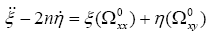(12)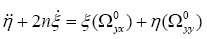(13)

Here, only linear terms in ξ and η have been taken. The second partial derivatives of Ω are denoted by subscripts x and y. The superscript 0 indicates that the derivatives are to be evaluated at the equilibrium point (x0, y0).

The characteristic equation corresponding to Equations (12) and (13):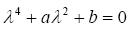(14)

where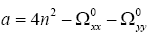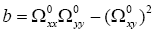Evaluating the second partial derivatives at the equilibrium points, we obtain: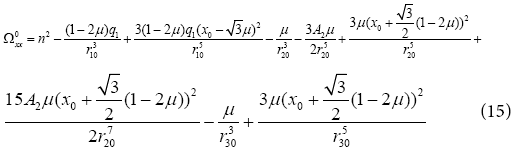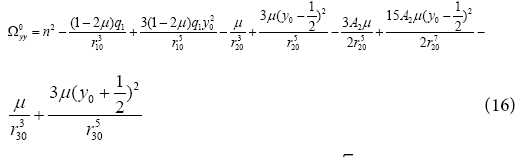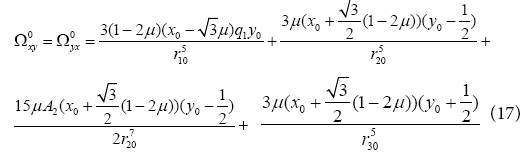with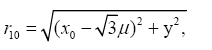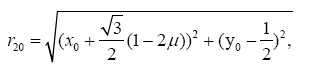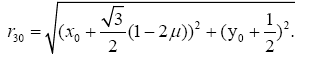The most fundamental questions about motion near the equilibrium points are those about the stability of these points. The stability of the non-collinear equilibrium points under the joint effects of radiation of the first primary and oblateness of the second primary are determined by the roots of the characteristic Equation (14). An equilibrium point will be stable if the Equation (14) evaluated at the equilibrium, has four pure imaginary roots or complex roots with negative real parts, otherwise it is unstable.

One can easily see that the given condition is equivalent to the following in equalities: being satisfied simultaneously.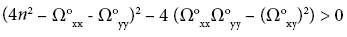(18)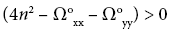(19)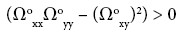(20)

We have computed the eigenvalues of the characteristic equation as the radiation and oblateness parameters varies with an arbitrary small steps and found that the equilibrium point L1 is unstable since for L1, the characteristic equation has eigenvalues of the form, λ1, 2,3,4 = ± a ± ib; L2, L3, L4, L5 and L6 are also unstable, since the eigenvalues are of the form, λ1,2= ± a, λ3,4= ± ib where a and b are real numbers, whereas, L7 and L8 are stable since the eigenvalues are of the form λ1,2= ±ia, λ3,4=±ib.

Discussion

We have studied the perturbed restricted four-body problem when the primary bodies m1 , m2 and m3 are always at the vertices of an equilateral triangle (Lagrangian configuration). The fourth particle in this system has negligible mass m with respect to the primaries, and its motion is perturbed by radiation pressure q1 and oblateness coefficient A2 from the primaries m1 and m2 , respectively. We studied the existence, location and stability of the equilibrium points as the radiation and oblateness parameters varies. The effects of the involved parameters on the positions of the equilibrium points are presented in Tables 1-3 and 4-6. These are shown graphically in Figures 3-6. It is found that for fixed values of oblateness coefficient A2 and decreasing values of radiation pressure q1 as well as for fixed values of radiation pressure q1 and increasing values of oblateness coefficient A2, positions of the equilibrium points are significantly affected.

Conclusion

Finally, the stability investigation has been achieved by determining the roots of the characteristic equation. The numerical investigation of these roots reveal that Li, i=1,...,6 are unstable due to the appearance of at least a positive root and/or a positive real part while L7 and L8 are stable due to the appearance of pure imaginary roots. It is remarkable to note that, equations of motion are unlike those obtained by Papadouris and Papadakis  due to the appearance of oblateness coefficient of the second small primary. We observe that the perturbation given in the oblateness coefficient which is considered permits the disappearance of collinear equilibrium points contrary to Kumari and Kushvah  results which has two collinear points. The positions of these points are different from those of Kumari and Kushvah .

ReferencesCan't read the image? click here to refresh